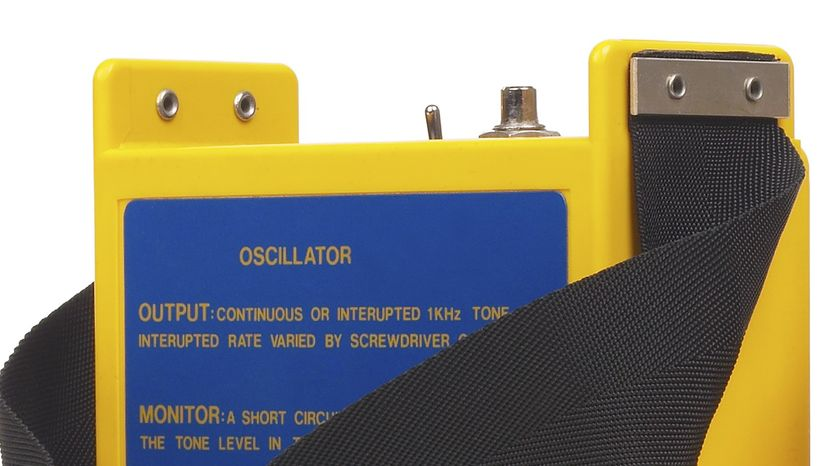How Oscillators WorkOscillators produce electronic signals. Hemera Technologies / Getty Images

Oscillators are important in many different types of electronic equipment. For example, a quartz watch uses a quartz oscillator to keep track of what time it is. An AM radio transmitter uses an oscillator to create the carrier wave for the station, and an AM radio receiver uses a special form of oscillator called a resonator to tune in a station. There are oscillators in computers, metal detectors and even stun guns.

­ To understand how electronic oscillators work, it is helpful to look at examples from the physical world. In this article, you'll learn the basic idea behind oscillators and how they're used in electronics.

­

Oscillation Basics

One of the most commonly used oscillators is the pendulum of a clock. If you push on a pendulum to start it swinging, it will oscillate at some frequency -- it will swing back and forth a certain number of times per second. The length of the pendulum is the main thing that controls the frequency.

For something to oscillate, energy needs to move back and forth between two forms. For example, in a pendulum, energy moves between potential energy and kinetic energy. When the pendulum is at one end of its travel, its energy is all potential energy and it is ready to fall. When the pendulum is in the middle of its cycle, all of its potential energy turns into kinetic energy and the pendulum is moving as fast as it can. As the pendulum moves toward the other end of its swing, all the kinetic energy turns back into potential energy. This movement of energy between the two forms is what causes the oscillation.

Eventually, any physical oscillator stops moving because of friction. To keep it going, you have to add a little bit of energy on each cycle. In a pendulum clock, the energy that keeps the pendulum moving comes from the spring. The pendulum gets a little push on each stroke to make up for the energy it loses to friction. See How Pendulum Clocks Work for details.

An electronic oscillator works on the same principle.

Oscillator CircuitsEnergy needs to move back and forth from one form to another for an oscillator to work. You can make a very simple oscillator by connecting a capacitor and an inductor together. If you've read How Capacitors Work and How Inductors Work, you know that both capacitors and inductors store energy. A capacitor stores energy in the form of an electrostatic field, while an inductor uses a magnetic field.

Imagine the following circuit:

If you charge up the capacitor with a battery and then insert the inductor into the circuit, here's what will happen:

• The capacitor will start to discharge through the inductor. As it does, the inductor will create a magnetic field.
• Once the capacitor discharges, the inductor will try to keep the current in the circuit moving, so it will charge up the other plate of the capacitor.
• Once the inductor's field collapses, the capacitor has been recharged (but with the opposite polarity), so it discharges again through the inductor.

­ This oscillation will continue until the circuit runs out of energy due to resistance in the wire. It will oscillate at a frequency that depends on the size of the inductor and the capacitor.

ResonatorsIn a simple crystal radio (see How Radio Works for details), a capacitor/inductor oscillator acts as the tuner for the radio. It is connected to an antenna and ground like this:

Thousands of sine waves from different radio stations hit the antenna. The capacitor and inductor want to resonate at one particular frequency. The sine wave that matches that particular frequency will get amplified by the resonator, and all of the other frequencies will be ignored.